T Predicting Variation on Void Ratio Deposition Influenced by Hydraulic Conductivity and Porosity Impact in Heterogeneous Silty and Peat Soil Formation|crimson publishers.com
• Submissions# Predicting Variation on Void Ratio Deposition Influenced by Hydraulic Conductivity and Porosity Impact in Heterogeneous Silty and Peat Soil Formation

Jaja GWT and Eluozo SN*

Department of Civil Engineering, Nigeria

*Corresponding author: Eluozo SN, Department of Civil Engineering, Faculty of Engineering, Nigeria

Submission: November 16, 2018;Published: November 27, 2018

DOI: 10.31031/ACET.2018.02.000546Volume2 Issue5
November 2018

#### Abstract

The study predicts the variation of void ratios on hydraulic conductivity and porosity impact for silty and peat sand deposition. The rate conductivity and porosity were considered in the system to predominantly pressure the deposition of void ratio on silty and peat sand formation, such structural deposition experienced predominant heterogeneity in the study location, the deposition reflected unconsolidated deposition that should be evaluated in fundamental analysis of engineering properties of soil for design of foundation, these condition made the study imperative, several experts may always apply the conventional system to generate parameters for void ratio, but the compressive analysis in most time are not gotten, but the analytical techniques applied were able to develop model that can determine the comprehensive deposition of void within the intercedes of the formation, the study has developed another conceptual approach to thoroughly monitor void ratio within silty and peat soil formation.

Keywords: Predicting void ratio hydraulic conductivity; Silty and peat sand

#### Introduction

current study on nature of soil formations and its engineering stress-strain response Indicate that the soil performs as a collection of scale-level-dependent skeletons arranged in a Particular manner [1-3]. However, several studies have mentioned that the physical nature of silty sand is entirely different from that of clean sand [1,3- 7]. They recognized that the undrained residual shear strength (Sus) response depends effectively on the void ratio as a state parameter. It is also anticipated that the global void ratio (e) cannot represent the amount of particle contacts in the sand-silt mixture samples [8- 12]. As the void ratio and proportion of the coarse-grained soil or fine-grained soil changes, the nature of their microstructures also changes [13,14]. Due to a large grain size distribution range and availability of voids larger than some grains, at low fines contents, some of the finer grains may remain inactive and swim in the void spaces without affecting or contributing to the force chain [15-17]. Therefore, it is quite important to use new index parameters such as the intergranular [2,18,19]

### Theoretical backgroundReplace n in the 1st term by n+2 and in the 2nd term by n+1, so that we have;Subject equation (16) to the following boundary conditionsSubstitute (18) into equation (17)Hence the particular solution of equation (16) is of the form:#### Materials and Method

Standard laboratory experiment where performed to monitor the void Ratio deposition at different formation, the soil deposition of the strata were collected in sequences base on the structural deposition at different study area, this samples collected at different location generate variation at different depth producing different deposition strata void ratio base on their litho structures the experimental result are applied to compare with theoretical values for model validation [20-22].

#### Result and Discussion

Results and discussion are presented in Table 1-10 including graphical representation of void ratio at different litho structures. Figure 1-10 shows the deposition of void ratios in linear structural setting as presented in the figures, the depositions of the void ratios within silty and peat formations explain the rate of heterogeneity in deposition of void within the intercede of the formation, the exponential setting from all the graphical representation express the rate of the litho structures of the soil in terms geomorphology effect and geochemistry in the strata depositions, the geological reflection of the location were also observed in the study to pressure the structural setting of the strata. The derived model solution were applied to monitor the depositions as in numerical and analytical setting through simulation, the study has observed the variation of the void from the generated predictive values thus compared with experimental values, it is concluded that the deposition of silty and peat soil in deltaic environment were based on the rate of heterogeneity level of disintegration of the predominant deposited porous rock in deltaic locations. The validation of the simulation developed favourable fits, the derived solution has predicted the void within silty and peat soil formation. The determination of void ratios is basic principle of engineering properties of soil for design of various foundation thus the rate consolidation including settlement of different condition in soil engineering.

Figure 1:Predictive values of void ratio at different depths.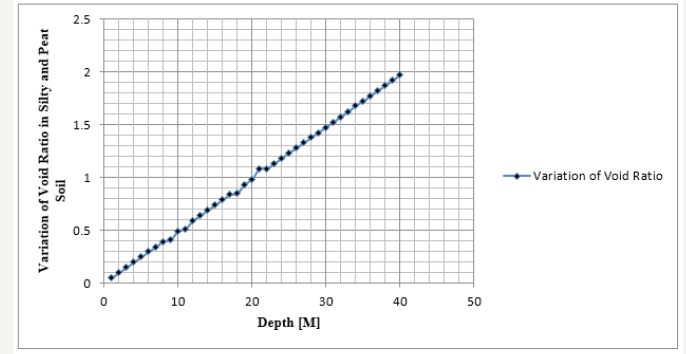Figure 2:Comparison of predictive and measured values of void ratio at different depthFigure 3:Comparison of predictive and measured values of void ratio at different depth.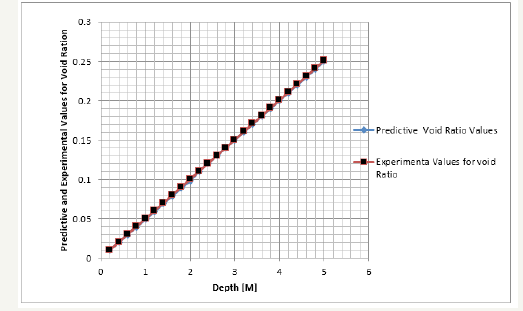Figure 4:Predictive values of void ratio at different depths.Figure 5:Predictive values of void ratio at different depths.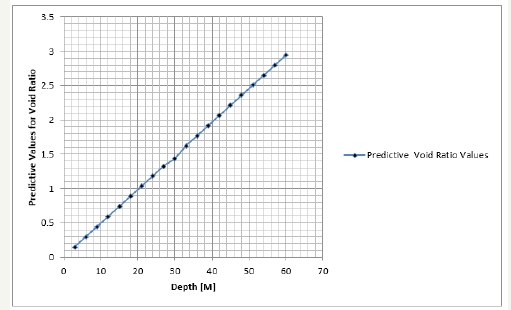Figure 6:Comparison of predictive and measured values of void ratio at different depth.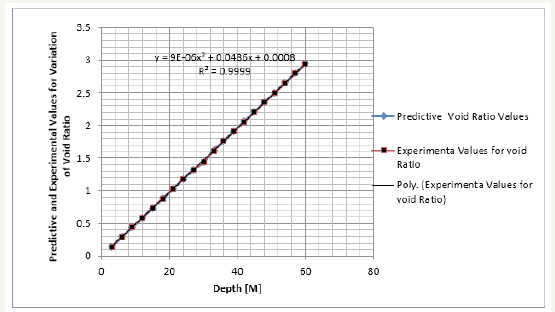Figure 7:Comparison of predictive and measured values of void ratio at different depth.Figure 8:Comparison of predictive and measured values of void ratio at different depth.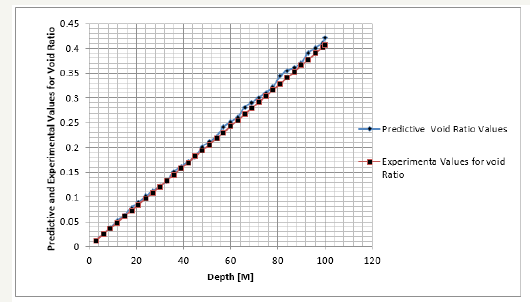Figure 9:Comparison of predictive and measured values of void ratio at different depth.Figure 10:Comparison of predictive and measured values of void ratio at different depth.Table 1:Predictive values of void ratio at different depthsTable 2:Comparison of predictive and measured values of void ratio at different depth.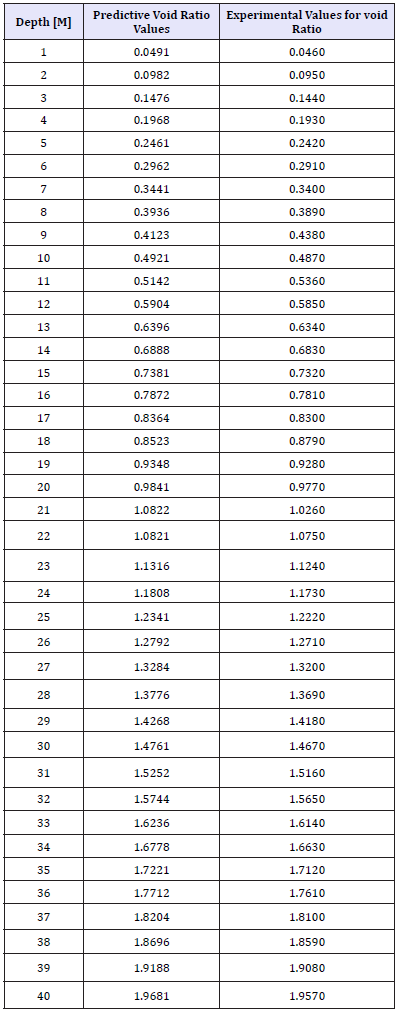Table 3:Comparison of predictive and measured values of void ratio at different depth.Table 4:Predictive values of void ratio at different depths.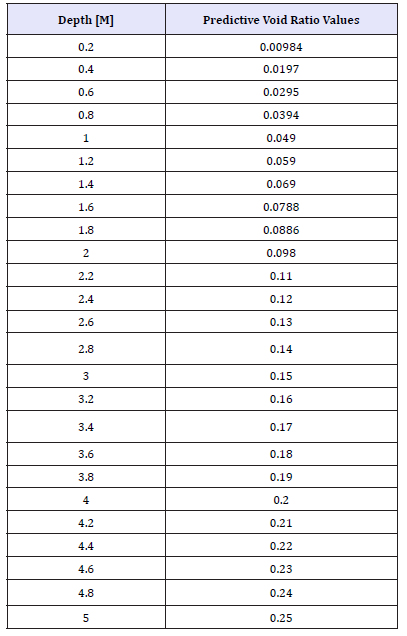Table 5:Predictive values of void ratio at different depths.Table 6:Comparison of predictive and measured values of void ratio at different depth.Table 7:Comparison of predictive and measured values of void ratio at different depth.Table 8:Comparison of predictive and measured values of void ratio at different depth.Table 9:Comparison of predictive and measured values of void ratio at different depth.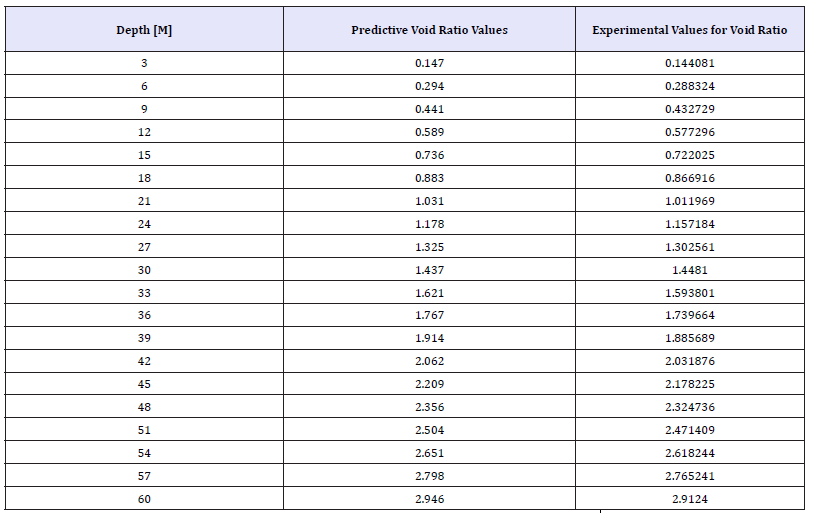Table 10:Comparison of predictive and measured values of void ratio at different depth.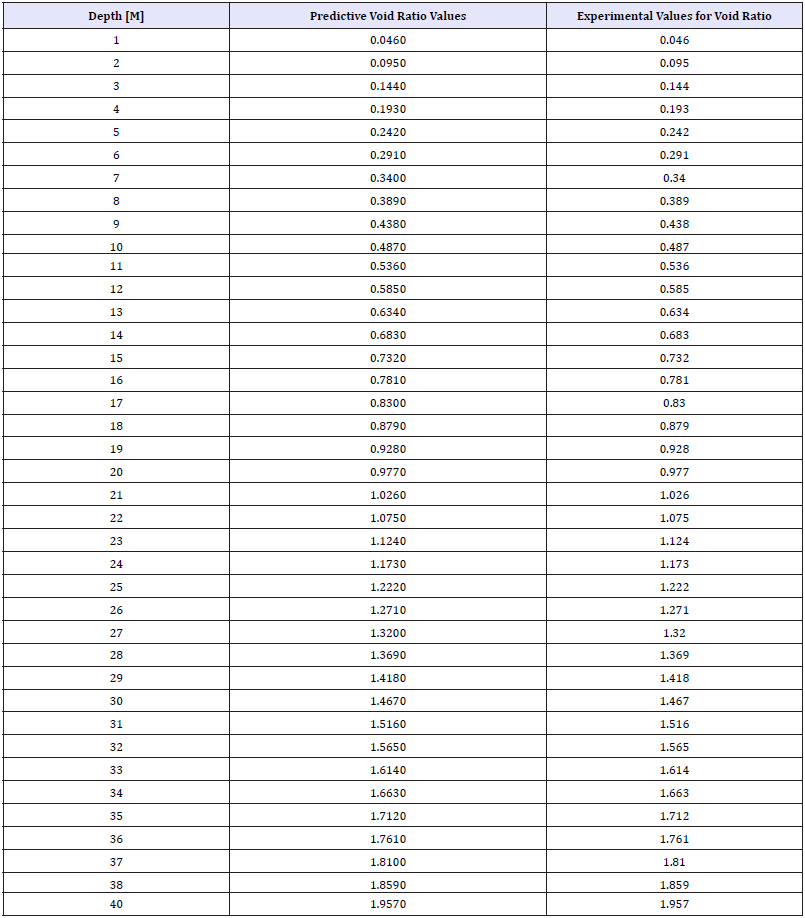#### Conclusion

The prediction of void ratios for silty and peat soil formation was to determine the heterogeneity of void ratios in peat and silty depositions under the influences of hydraulic conductivities and porosities in silty and peat sand depositions. The study tries to predict the structural deposition of silty and peat through their disintegration from predominant porous rock in deltaic environment, from the graphical representations, it was observed that the structures of the formations experiences linear depositions from the made soil to peat soil. Void ratios were in heterogeneity in exponential setting observed from graphical representations, this implies that the litho structures were influenced by the level of disintegration in the porous rock under the influences from variations of porosities and hydraulic conductivities, the derived solution were subjected to simulation, the validation generated favourable fits, these explain the void ratios within silty and peat soil depositions in deltaic environment. The basic principles in engineering properties of soil mechanics has been developed applying this analytical or deterministic modelling techniques. This can be applied to determine void ratios in settlements or any other design of foundation system.

We at Crimson Publishing are a group of people with a combined passion for science and research, who wants to bring to the world a unified platform where all scientific know-how is available read more...